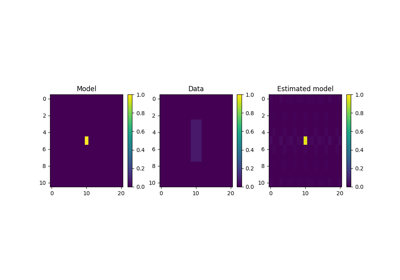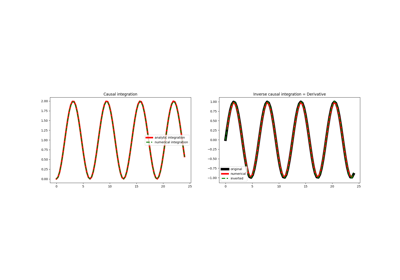# pylops.Smoothing2D¶

pylops.Smoothing2D(nsmooth, dims, nodir=None, dtype='float64')[source]

2D Smoothing.

Apply smoothing to model (and data) along two directions of a multi-dimensional array depending on the choice of nodir.

Parameters: nsmooth : Lenght of smoothing operatorin 1st and 2nd dimensions (must be odd) dims : tuple Number of samples for each dimension nodir : int, optional Direction along which smoothing is NOT applied (set to None for 2d arrays) dtype : str, optional Type of elements in input array.

lops.signalprocessing.Convolve2D
2D convolution

Notes

The 2D Smoothing operator is a special type of convolutional operator that convolves the input model (or data) with a constant 2d filter of size $$n_{smooth, 1} \quad x \quad n_{smooth, 2}$$:

Its application to a two dimensional input signal is:

$y[i,j] = 1/(n_{smooth, 1}*n_{smooth, 2}) \sum_{l=-(n_{smooth,1}-1)/2}^{(n_{smooth,1}-1)/2} \sum_{m=-(n_{smooth,2}-1)/2}^{(n_{smooth,2}-1)/2} x[l,m]$

Note that since the filter is symmetrical, the Smoothing2D operator is self-adjoint.

Attributes: shape : tuple Operator shape explicit : bool Operator contains a matrix that can be solved explicitly (True) or not (False)

## Examples using pylops.Smoothing2D¶2D SmoothingCausal Integration